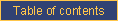homefeaturesexamplesDOCUMENTATIONblogpricingcontact ustechnical documentationCATI documentationcookbookrobotEnsuring that category permutations are the same for two questions

Problem

You want the answer categories of two questions to be presented in random order but in the same order for both questions.

Solution

Use the ROTATION=Qx feature to create parallel permutations.

Discussion

Say, in question Q1, you are asking whether one has read each magazine in a list over the past month. You want that list to be presented in random order to avoid order effects. You can simply add the ROTATION keyword to the question name line.

Let's add a second question Q2 which uses the same list; you want the order of presentation of magazines in Q2 to be the same as in Q1. Then use ROTATION=Q1 on the Q2 question line. This indicates that the answer categories for Q2 should be in the same order as those in Q1.

If Q1 and Q2 are in a question permutation, it is possible that CallWeb would display Q2 before Q1. In such circumstance, there would be no random order to apply to the answer categories in Q2 and CallWeb would generate an answer category permutation for Q2 independently from Q1. In this example, to make sure that Q1 and Q2 use parallel permutation, use ROTATION=Q2 for Q1 and ROTATION=Q1 for Q2. The first question displayed will control the persentation of the answer categories of the second one.

This recipe applies to two questions. If more than two questions must share a random order of answer caregories, make sure that one of them is always displayed first and make the other ones dependent upon that first question.e-mail technical support!